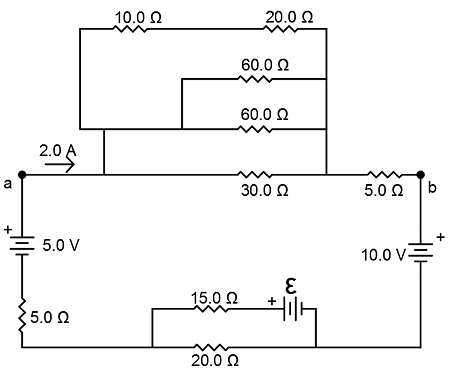# Consider the circuit shown. Image src='circuit3057649903852599820.png' alt='' caption='Figure' ...

## Question:

Consider the circuit shown.A.) Find the total equivalent resistance between {eq}\displaystyle a {/eq} and {eq}\displaystyle b {/eq}.

B.) Find the current through the {eq}\displaystyle 20 \ \Omega {/eq} resistor (at the bottom of the circuit). Specify the direction.

C.) Find the current through the {eq}\displaystyle 15 \ \Omega {/eq} resistor. Specify the direction.

D.) Find the unknown emf {eq}\displaystyle \xi {/eq}.

## Parallel Linear Circuits:

The equivalent resistance in linear circuits is such that the reciprocal of the equivalent resistance is equal to the sum of the reciprocals of the parallel circuits. The potential difference of parallel paths is the same.

#### Part A

Using the symbol || to denote a parallel connection and the symbol + to mean simple addition in a series connection, we determine the...

Become a Study.com member to unlock this answer! Create your account